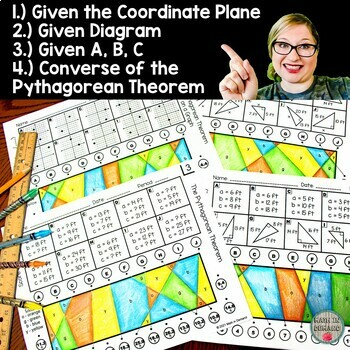# The Pythagorean Theorem Stained Glass Activities

Rated 5 out of 5, based on 1 reviews
1 Rating;
8th - 9th
Subjects
Resource Type
Formats Included
• PDF
Pages
Report this resource to TPT
##### Also included in
1. This is a bundle for all of my 8th Grade Math Stained Glass Activities. This bundle includes the following math concepts:1.) Solving Two-Step Equations2.) Solving Two-Step Equations with Fractions3.) Solving Two-Step Equations with Parentheses4.) Solving Equations with Variables on Both Sides5.) Slo
Price \$22.50Original Price \$25.00Save \$2.50

### Description

There are 4 worksheets on the Pythagorean Theorem. The first worksheet includes calculating the distance between two points on a coordinate plane by using the Pythagorean Theorem. The second worksheet shows diagrams of right triangles. The third worksheet gives A, B, or C. The fourth worksheet is on the Converse of the Pythagorean Theorem. Each worksheet contains 10 problems.

These worksheets help with self-assessment due to creating a stained glass look. Students will use a ruler or straight edge to connect the letter of the question to its answer. After connecting 10 lines, students should see a letter in each section. Students will color each section according to the color code.

Total Pages